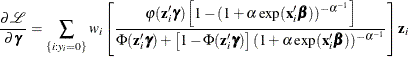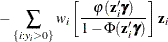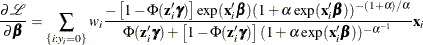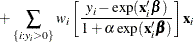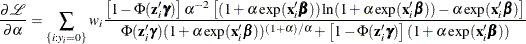### Zero-Inflated Negative Binomial Regression

The zero-inflated negative binomial (ZINB) model in PROC TCOUNTREG is based on the negative binomial model with quadratic variance function (p=2). The ZINB model is obtained by specifying a negative binomial distribution for the data generation process referred to earlier as Process 2: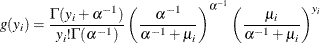Thus the ZINB model is defined to be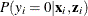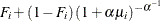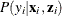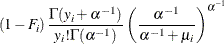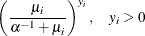In this case, the conditional expectation and conditional variance ofare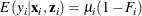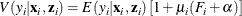As with the ZIP model, the ZINB model exhibits overdispersion because the conditional variance exceeds the conditional mean.

#### ZINB Model with Logistic Link Function

In this model, the probability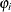is given by the logistic function—namely,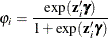The log-likelihood function is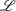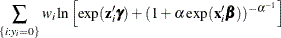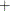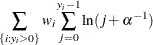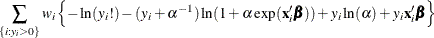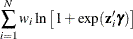See the section Poisson Regression for the definition of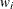.

The gradient for this model is given by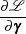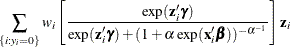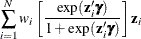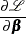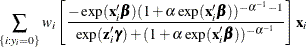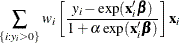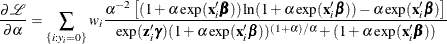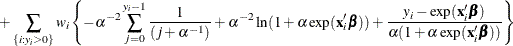#### ZINB Model with Standard Normal Link Function

For this model, the probabilityis specified with the standard normal distribution function (probit function):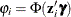. The log-likelihood function is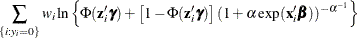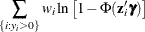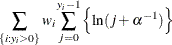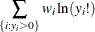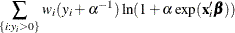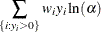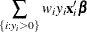See the section Poisson Regression for the definition of.

The gradient for this model is given by